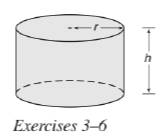Chapter 9.3, Problem 3E### Elementary Geometry for College St...

6th Edition
Daniel C. Alexander + 1 other
ISBN: 9781285195698

#### Solutions

Chapter
Section### Elementary Geometry for College St...

6th Edition
Daniel C. Alexander + 1 other
ISBN: 9781285195698
Textbook Problem
1 views

# For the right circular cylinder shown on page 471, r = 3.2 cm and h = 5.1 cm. Find the approximate volume of the cylinder.To determine

To calculate:

The approximate volume of the cylinder.

Explanation

Given:

For a right circular cylinder r=3.2cm and h=5.1cm.

Formula used:

The volume of the right circular cylinder is V=πr2h.

Calculation:

Substitute the known values in the formula,

V=πr2h=π(3

### Still sussing out bartleby?

Check out a sample textbook solution.

See a sample solution

#### The Solution to Your Study Problems

Bartleby provides explanations to thousands of textbook problems written by our experts, many with advanced degrees!

Get Started

#### Let h be the function defined by h(x) = x3 x2 + x + 1. Find h(5), h(0), h(a), and h(a).

Applied Calculus for the Managerial, Life, and Social Sciences: A Brief Approach

#### True or False: If f′(c) = 0 and f″(c) < 0, then c is a local minimum.

Study Guide for Stewart's Single Variable Calculus: Early Transcendentals, 8th

#### Graph each function. fx=2+lnx

College Algebra (MindTap Course List)